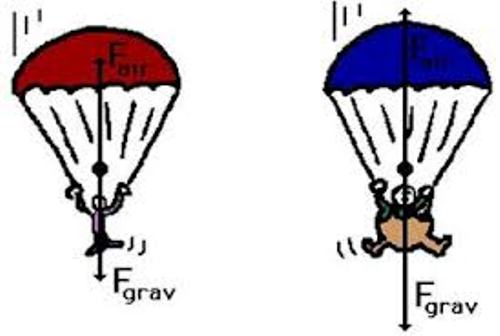# Resultant Forces and Work Done

?
• Created by: Davwi
• Created on: 19-02-19 05:22

Free body diagrams

• The sizes of the arrows show the magnitude of the force.
• The directions show the direction of the force.
• A free body diagram only incorporates forces exerted on an object and not the force the object exerts to its surroundings.
• Here is an example:• In this example you can see the different amounts of gravity and air resistance that contribute to a drop.

Resultant Force

• In most real situations, there are at least 2 forces acting on an object.
• If you have a certain number of forces acting on a single object, you can replace them with a single force, so long as this single force as the same effect as all the forces added together.
• This is called the resultant force.
• If the forces all act in the same direction, then you add them to get your resultant force, and subtract any going in the opposite direction.
• When a force moves through a distance, energy is transferred, therefore work is done.
• To make something move, or keep it moving in the case of frictional forces, a force must be applied.
• The thing applying the force needs a source of energy.
• The force does work to move the object and energy is transferred from one store to…